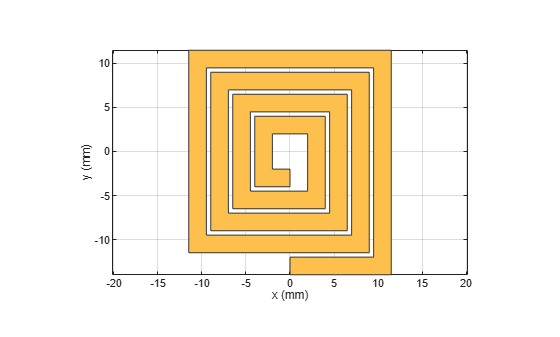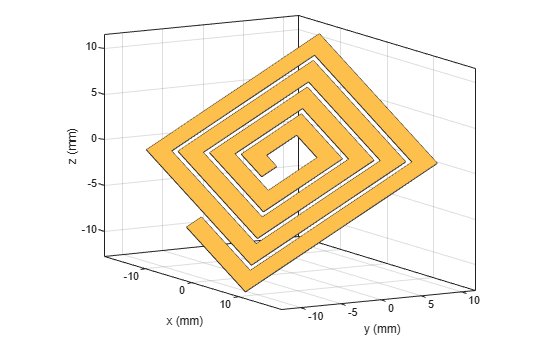rotate

Rotate RF PCB shape about defined axis

Description

example

c = rotate(shape,angle,axis1,axis2) rotates a shape by a specified angle about an axes defined by two points axis1 and axis2.

Examples

collapse all

Create and display a spiral trace.

trace = traceSpiral;
show(trace)Specify two points that define the axes of rotation.

pt1 = [0 0 0];
pt2 = [1 1 0];

Rotate the spiral trace by 45 degrees about the axis.

traceRot = rotate(trace,45,pt1,pt2);

Display the rotated shape. Set the camera line of sight to display in 3-D space.

show(traceRot)
view(60,10)Input Arguments

collapse all

RF PCB shape created using custom elements and shape objects of RF PCB Toolbox™, specified as an object.

Example: shape = bendCurved; specifies the shape as a bendCurved object.

Angle of rotation, specified as a scalar in degrees.

Example: 45 rotates the shape around the axis by 45 degrees.

Data Types: double

One point on the axis of rotation, specified as a three-element vector of Cartesian coordinates in meters.

Example: [0 0 0]

Data Types: double

Second point on the axis of rotation, specified as a three-element vectors of Cartesian coordinates in meters. axis2 must be different than axis1.

Example: [0 0 1]

Data Types: double